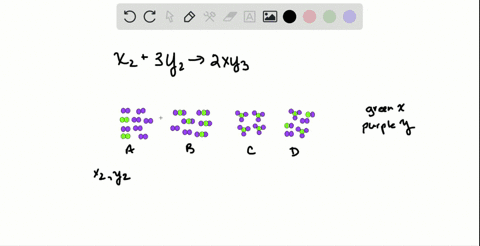🎉 The Study-to-Win Winning Ticket number has been announced! Go to your Tickets dashboard to see if you won! 🎉View Winning Ticket### Elements $X(\text {green})$ and $\mathrm{Y}$ (pur…

01:20University of Maine
Problem 119

# Calculate each of the following quantities:(a) Amount (mol) of 0.588 g of ammonium bromide(b) Number of potassium ions in 88.5 $\mathrm{g}$ of potassium nitrate(c) Mass (g) of 5.85 mol of glycerol $\left(\mathrm{C}_{3} \mathrm{H}_{8} \mathrm{O}_{3}\right)$(d) Volume (L) of 2.85 $\mathrm{mol}$ of chloroform $\left(\mathrm{CHCl}_{3} ; d=1.48 \mathrm{g} / \mathrm{mL}\right)$(e) Number of sodium ions in 2.11 mol of sodium caa(f) Number of atoms in 25.0$\mu \mathrm{g}$ of cadmium(g) Number of atoms in 0.0015 mol of fluorine gas

## Discussion

You must be signed in to discuss.